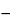Aluminum Dichlorohydrate Solution
» Aluminum Dichlorohydrate Solution consists of complex basic aluminum chloride that is polymeric and encompasses a range of aluminum-to-chloride atomic ratios between 0.90:1 and 1.25:1. The following solvents may be used: water, propylene glycol, dipropylene glycol, or alcohol. It contains the equivalent of not less than 90.0 percent and not more than 110.0 percent of the labeled concentration of anhydrous aluminum dichlorohydrate.
Packaging and storage— Preserve in well-closed containers.
Labeling— Label Solution to state the solvent used and the claimed concentration of anhydrous aluminum dichlorohydrate contained therein.
Identification—
Test specimen— Add about 10 mL of isopropyl alcohol to 2 g of Solution, mix, and filter. Evaporate the filtrate to about 1 mL on a steam bath. Deposit this solution on a silver chloride disk.
Standard specimen: a similar preparation of propylene glycol.
Test specimen— Add about 10 mL of isopropyl alcohol to 2 g of Solution, mix, and filter. Evaporate the filtrate to about 1 mL on a steam bath. Deposit this solution on a silver chloride disk.
Standard specimen: a similar preparation of dipropylene glycol.
D: Identification of alcohol (where stated on the label)—Mix 5 drops of Solution in a small beaker with 1 mL of potassium permanganate solution (1 in 100) and 5 drops of 2 N sulfuric acid, and cover the beaker immediately with filter paper moistened with a freshly prepared solution of 0.1 g of sodium nitroferricyanide and 0.25 g of piperazine in 5 mL of water: an intense blue color is produced on the filter paper, the color becoming paler after a few minutes.
between 3.0 and 5.0, in a solution prepared by diluting 3 g of the Solution with water to obtain 10 mL.
Prepare the Test Preparation using an accurately weighed quantity of the Solution. The limit is 2 µg per g.
Prepare the Test Preparation using an accurately weighed quantity of the Solution. The limit is 10 µg per g.
Limit of iron— Using Aluminum Dichlorohydrate Solution instead of Aluminum Chlorohydrate Solution, proceed as directed in the test for the Limit of iron under Aluminum Chlorohydrate Solution. The limit is 75 µg per g.
Content of aluminum— Using Aluminum Dichlorohydrate Solution instead of Aluminum Chlorohydrate Solution, proceed as directed in the test for the Content of aluminum under Aluminum Chlorohydrate Solution. Use the result to calculate the Aluminum/chloride atomic ratio.
Content of chloride— Using Aluminum Dichlorohydrate Solution instead of Aluminum Chlorohydrate Solution, proceed as directed in the test for the Content of chloride under Aluminum Chlorohydrate Solution. Use the result to calculate the Aluminum/chloride atomic ratio.
Aluminum/chloride atomic ratio— Divide the percentage of aluminum found in the test for Content of aluminum by the percentage of chloride found in the test for Content of chloride, and multiply by 35.453/26.98, in which 35.453 and 26.98 are the atomic weights of chlorine and aluminum respectively: the ratio is between 0.90:1 and 1.25:1.
Assay— Calculate the percentage of anhydrous aluminum dichlorohydrate in the Solution by the formula:
Al({26.98x + [17.01(3x1)] + 35.453} / 26.98x)
in which Al is the percentage of aluminum found in the test for Content of aluminum, x is the aluminum/chloride atomic ratio found in the test for aluminum/chloride atomic ratio, 26.98 is the atomic weight of aluminum, 17.01 is the molecular weight of the hydroxide anion (OH), and 35.453 is the atomic weight of chlorine (Cl).
Auxiliary Information— Please check for your question in the FAQs before contacting USP.
Topic/Question Contact Expert Committee
Monograph Feiwen Mao, M.S.
Senior Scientific Liaison
1-301-816-8320
(SM32010) Monographs - Small Molecules 3
USP35–NF30 Page 2130